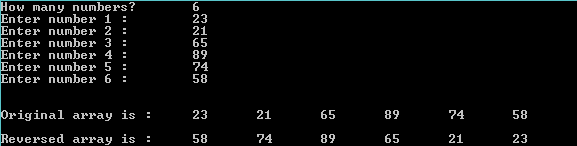## Friday, 9 January 2015

### C Program to Reverse all the elements in an array

This is a C Program which reverses all the elements present in an array.

Here we read  all the elements from the array and again read them from last element and print them back.

PROGRAM :
```#include <stdio.h>
#include <stdlib.h>

int main()
{
int i,n,a,b,c,d;
printf("How many numbers? \t");
scanf("%d",&n);
for(i=0;i<n;i++)
{
printf("Enter number %d : \t",i+1);
scanf("%d",&a[i]);
}
printf("\n\nOriginal array is :\t");
for(i=0;i<n;i++)
{
printf("%d \t",a[i]);
}

for (c = n - 1, d = 0; c >= 0; c--, d++)
b[d] = a[c];

for (c = 0; c < n; c++)
a[c] = b[c];

printf("\n\nReversed array is :\t");

for (c = 0; c < n; c++)
printf("%d\t", a[c]);
return 0;
}```

OUTPUT :C - Reverse the elements in an array